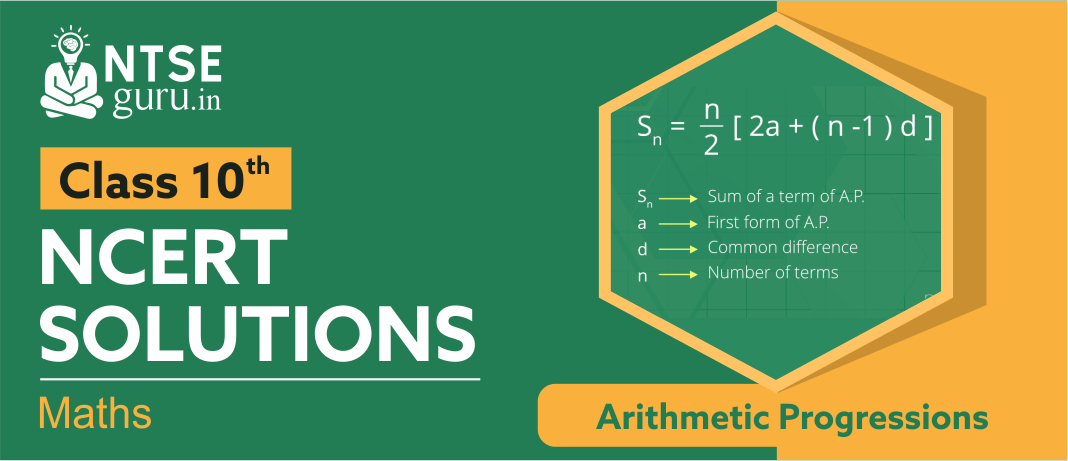# NCERT Solutions For Class 10 Maths Chapter 5An arithmetic progression (AP), also called an arithmetic sequence, is a sequence of numbers which differ from each other by a common difference. For example, the sequence. 2, 4, 6, 8, is an arithmetic sequence with the common difference 2. NCERT Solutions for Class 10 Maths Chapter 5, ‘Arithmetic Progressions’  explains students on the most basic and the oldest part of mathematics that deals with rules for basic operation on numbers including counting, addition, subtraction, multiplication and division.

## Arithmetic Progressions - Class 10 NCERT Solutions

When you are waiting for a bus. Assuming that the traffic is moving at a constant speed you can predict when the next bus will come. If you ride a taxi, you will be charged an initial rate and then a per mile or per kilometer charge that also has an arithmetic sequence. Given below are questions and answers on ‘Arithmetic Progressions - Class 10 NCERT Solutions’ for your reference.

For more such chapter-wise solutions, subject sample papers, previous year questions, and practice sheets you can log in to our website www.ntseguru.in or can download our ntse guru mobile app for free on your android phone from play store.